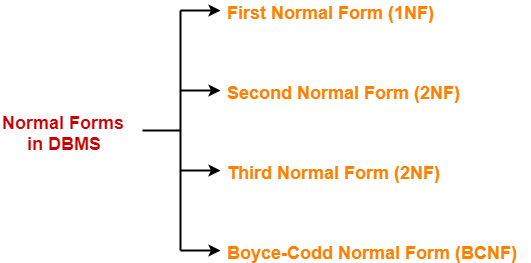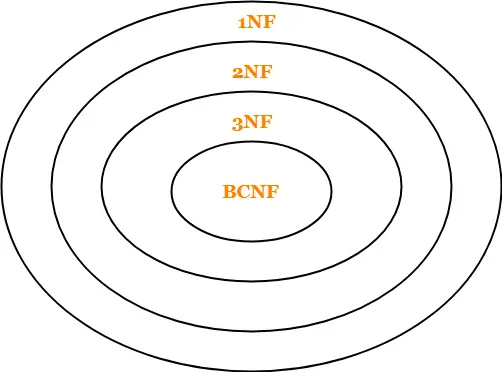## Normal Forms in DBMS-

Before you go through this article, make sure that you have gone through the previous article on Normalization in DBMS.

We have discussed-

• Database normalization is a process of making the database consistent.
• Normalization is done through normal forms.
• The standard normal forms generally used are-## Point-01:

Remember the following diagram which implies-

• A relation in BCNF will surely be in all other normal forms.
• A relation in 3NF will surely be in 2NF and 1NF.
• A relation in 2NF will surely be in 1NF.## Point-02:

The above diagram also implies-

• BCNF is stricter than 3NF.
• 3NF is stricter than 2NF.
• 2NF is stricter than 1NF.

## Point-03:

While determining the normal form of any given relation,

• Start checking from BCNF.
• This is because if it is found to be in BCNF, then it will surely be in all other normal forms.
• If the relation is not in BCNF, then start moving towards the outer circles and check for other normal forms in the order they appear.

## Point-04:

• In a relational database, a relation is always in First Normal Form (1NF) at least.

## Point-05:

• Singleton keys are those that consist of only a single attribute.
• If all the candidate keys of a relation are singleton candidate keys, then it will always be in 2NF at least.
• This is because there will be no chances of existing any partial dependency.
• The candidate keys will either fully appear or fully disappear from the dependencies.
• Thus, an incomplete candidate key will never determine a non-prime attribute.

Also read- Types of Keys in DBMS

## Point-06:

• If all the attributes of a relation are prime attributes, then it will always be in 2NF at least.
• This is because there will be no chances of existing any partial dependency.
• Since there are no non-prime attributes, there will be no Functional Dependency which determines a non-prime attribute.

## Point-07:

• If all the attributes of a relation are prime attributes, then it will always be in 3NF at least.
• This is because there will be no chances of existing any transitive dependency for non-prime attributes.

## Point-08:

• Third Normal Form (3NF) is considered adequate for normal relational database design.

## Point-09:

• Every binary relation (a relation with only two attributes) is always in BCNF.

## Point-10:

• BCNF is free from redundancies arising out of functional dependencies (zero redundancy).

## Point-11:

• A relation with only trivial functional dependencies is always in BCNF.
• In other words, a relation with no non-trivial functional dependencies is always in BCNF.

## Point-12:

• BCNF decomposition is always lossless but not always dependency preserving.

## Point-13:

• Sometimes, going for BCNF may not preserve functional dependencies.
• So, go for BCNF only if the lost functional dependencies are not required else normalize till 3NF only.

## Point-14:

• There exist many more normal forms even after BCNF like 4NF and more.
• But in the real world database systems, it is generally not required to go beyond BCNF.

## Point-15:

• Lossy decomposition is not allowed in 2NF, 3NF and BCNF.
• So, if the decomposition of a relation has been done in such a way that it is lossy, then the decomposition will never be in 2NF, 3NF and BCNF.

## Point-16:

• Unlike BCNF, Lossless and dependency preserving decomposition into 3NF and 2NF is always possible.

## Point-17:

• A prime attribute can be transitively dependent on a key in a 3NF relation.
• A prime attribute can not be transitively dependent on a key in a BCNF relation.

## Point-18:

• If a relation consists of only singleton candidate keys and it is in 3NF, then it must also be in BCNF.

## Point-19:

• If a relation consists of only one candidate key and it is in 3NF, then the relation must also be in BCNF.

Next Article- Transactions in DBMS

Get more notes and other study material of Database Management System (DBMS).

Watch video lectures by visiting our YouTube channel LearnVidFun.

## Normalization in DBMS-

In DBMS, database normalization is a process of making the database consistent by-

• Reducing the redundancies
• Ensuring the integrity of data through lossless decomposition

Normalization is done through normal forms.

## Normal Forms-

The standard normal forms used are-1. First Normal Form (1NF)
2. Second Normal Form (2NF)
3. Third Normal Form (3NF)
4. Boyce-Codd Normal Form (BCNF)

There exists several other normal forms even after BCNF but generally we normalize till BCNF only.

## First Normal Form-

A given relation is called in First Normal Form (1NF) if each cell of the table contains only an atomic value.

OR

A given relation is called in First Normal Form (1NF) if the attribute of every tuple is either single valued or a null value.

### Example-

The following relation is not in 1NF-

 Student_id Name Subjects 100 Akshay Computer Networks, Designing 101 Aman Database Management System 102 Anjali Automata, Compiler Design

Relation is not in 1NF

However,

• This relation can be brought into 1NF.
• This can be done by rewriting the relation such that each cell of the table contains only one value.

 Student_id Name Subjects 100 Akshay Computer Networks 100 Akshay Designing 101 Aman Database Management System 102 Anjali Automata 102 Anjali Compiler Design

Relation is in 1NF

This relation is in First Normal Form (1NF).

### NOTE-

• By default, every relation is in 1NF.
• This is because formal definition of a relation states that value of all the attributes must be atomic.

Second Normal Form-

A given relation is called in Second Normal Form (2NF) if and only if-

1. Relation already exists in 1NF.
2. No partial dependency exists in the relation.

Also read- Functional Dependency in DBMS

### Partial Dependency

A partial dependency is a dependency where few attributes of the candidate key determines non-prime attribute(s).

OR

A partial dependency is a dependency where a portion of the candidate key or incomplete candidate key determines non-prime attribute(s).

In other words,

A → B is called a partial dependency if and only if-

1. A is a subset of some candidate key
2. B is a non-prime attribute.

If any one condition fails, then it will not be a partial dependency.

### NOTE-

• To avoid partial dependency, incomplete candidate key must not determine any non-prime attribute.
• However, incomplete candidate key can determine prime attributes.

## Example-

Consider a relation- R ( V , W , X , Y , Z ) with functional dependencies-

VW → XY

Y → V

WX → YZ

The possible candidate keys for this relation are-

VW , WX , WY

From here,

• Prime attributes = { V , W , X , Y }
• Non-prime attributes = { Z }

Now, if we observe the given dependencies-

• There is no partial dependency.
• This is because there exists no dependency where incomplete candidate key determines any non-prime attribute.

Thus, we conclude that the given relation is in 2NF.

Third Normal Form-

A given relation is called in Third Normal Form (3NF) if and only if-

1. Relation already exists in 2NF.
2. No transitive dependency exists for non-prime attributes.

### Transitive Dependency

A → B is called a transitive dependency if and only if-

1. A is not a super key.
2. B is a non-prime attribute.

If any one condition fails, then it is not a transitive dependency.

### NOTE-

• Transitive dependency must not exist for non-prime attributes.
• However, transitive dependency can exist for prime attributes.

OR

A relation is called in Third Normal Form (3NF) if and only if-

Any one condition holds for each non-trivial functional dependency A → B

1. A is a super key
2. B is a prime attribute

## Example-

Consider a relation- R ( A , B , C , D , E ) with functional dependencies-

A → BC

CD → E

B → D

E → A

The possible candidate keys for this relation are-

A , E , CD , BC

From here,

• Prime attributes = { A , B , C , D , E }
• There are no non-prime attributes

Now,

• It is clear that there are no non-prime attributes in the relation.
• In other words, all the attributes of relation are prime attributes.
• Thus, all the attributes on RHS of each functional dependency are prime attributes.

Thus, we conclude that the given relation is in 3NF.

## Boyce-Codd Normal Form-

A given relation is called in BCNF if and only if-

1. Relation already exists in 3NF.
2. For each non-trivial functional dependency A → B, A is a super key of the relation.

## Example-

Consider a relation- R ( A , B , C ) with the functional dependencies-

A → B

B → C

C → A

The possible candidate keys for this relation are-

A , B , C

Now, we can observe that RHS of each given functional dependency is a candidate key.

Thus, we conclude that the given relation is in BCNF.

Next Article- Important Points About Normal Forms

Get more notes and other study material of Database Management System (DBMS).

Watch video lectures by visiting our YouTube channel LearnVidFun.